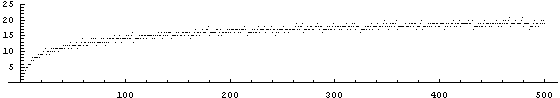## Integer Complexity

Ed Pegg Jr., April 12, 2004

Using the set of symbols {1, ×, +} and parentheses, how many 1's does it take to represent 23?  It turns out that eleven are needed.  Surprisingly, the number 27 requires only nine.

23 = (1+1)×((1+1)×((1+1)×(1+1)+1)+1)+1
27 = (1+1+1) × (1+1+1) × (1+1+1)

The first 15 numbers, in their representations with the fewest 1's, are as follows: 1,  1+1,  1+1+1,  (1+1)×(1+1),  (1+1)×(1+1)+1,  (1+1)×(1+1+1),  (1+1)×(1+1+1)+1,  (1+1)×(1+1)×(1+1),  (1+1+1)×(1+1+1),  (1+1)×((1+1)×(1+1)+1),  (1+1)×((1+1)×(1+1)+1)+1,  (1+1+1)×(1+1)×(1+1),  (1+1+1)×(1+1)×(1+1)+1,  (1+1)×((1+1)×(1+1+1)+1), and (1+1+1)×((1+1)×(1+1)+1).  The number of ones needed gives the sequence {1, 2, 3, 4, 5, 5, 6, 6, 6, 7, 8, 7, 8, 8, 8, ...}, or sequence A005245 in the Encyclopedia of Integer Sequences.Figure 1. Sequence A005245 in the Encyclopedia of Integer Sequences.

You might notice that all prime numbers are the previous number +1, and that all non-primes are products of lower primes. It is easy to find a "complexity" by this method, since it involves only factoring.  For example, 61993 → 47×1319 → (46+1)×(1312+1) → ((2×23)+1)×((2×659)+1) → ((2×(22+1))+1)×((2×(658+1))+1)→ ((2×((2×11)+1))+1)×((2×((2×7×47)+1))+1), and so on. This method gives the optimal presentation for all numbers until 46 is reached.  Then it starts failing.

46 = (1+1)×((1+1)×((1+1)×((1+1)×(1+1)+1)+1)+1)  -- using 2×23 -- needs 13 1's.
46 = (1+1+1)×(1+1+1)×((1+1)×(1+1)+1)+1 -- using 3×3×5+1 -- only needs 12 1's.

This process of subtracting one from primes and factoring nonprimes gives an upper bound for A005245, and is represented as A061373. There was a conjecture listed within A005245 that 0 ≤ A061373(n) - A005245(n) ≤ 1, which I thought might be nice to present here as a problem to solve.  Alas, I easily disproved it myself.  The conjecture fails at 235.

235 = ((1+1)×(1+1)+1)×((1+1)×((1+1)×((1+1)×((1+1)×(1+1)+1)+1)+1)+1)  -- using 5×47 -- needs 19 1's
235 = (1+1)×(1+1+1)×(1+1+1)×((1+1+1)×(1+1)×(1+1)+1) -- using 2×3×3×13+1 -- only needs 17 1's.

The numbers {46, 235, 649, 1081, 7849, 31669, 61993} require {1,2,3,4,5,6,7} less 1's in A005245 than in A061373.  Out of the first 150000 numbers, the two sequences require the same number of 1's 54000 times.  Although the first ten million terms are known, a good function for determining A005245 remains undiscovered.  First values with a given complexity are {1, 2, 3, 4, 5, 7, 10, 11, 17, 22, 23, 41, ...}, or sequence A005520.  The last eight known values of A005520 are equal to -1 mod 120.  Does this happen for all higher terms?  Final values with a given complexity are {1, 2, 3, 4, 6, 9, 12, 18, 27, 36, 54, 81, ...} or sequence A000792.  There are many unanswered questions.

A natural extension of this is to consider the set of symbols {i, ×, +}, extending the problem into complex numbers.  The below picture gives a map of the complexity -- that is, the necessary number of i's -- for complex integers -100-100i to 100+100i.  Note that 0, at the center, requires 4 i's (i + i×i×i).  The darker the pixel, the greater the complexity.  This is a rather tangled web, with all sorts of patterns.Figure 2.  The complexity of the complex integers.

For his book A New Kind of Science (now an online reference), Stephen Wolfram considered the integer complexity problem in a variety of ways.  For example, the symbol ◦ can be defined in many ways.  What if ◦ is defined so that a◦b = 2×a + b - 1? Thusly defined, 7◦13 = 2×7 + 13 - 1 = 26.  If we have the set of symbols {1, ◦}, it turns out any positive integer can be represented under this definition.  For example, 6 = (1◦(1◦1))◦1.  This complexity measure happens to match sequence A056792 {1,2,3,3,4,4,5,4,5,5,6,5,6,6,7,5,6,6,7, ...}. A056792 = binary length + binary weight - 1.  For example, 61993 has binary representation 1111001000101001, with length 16 and weight 8, so the complexity of 61993 is 16+8-1 = 23.Figure 3. Sequence A056792 in the Online Encyclopedia of Integer Sequences.

NKS examined several other representations for {1, ◦}, with various definitions of ◦, that will produce all positive integers.  (See NKS online page 916).Figure 4. Define a◦b = BitXor[2a,b]+1. {1, 6, 5, 2, 5, 4, 3, 6, 4, 3, 5, 5, 4, 5, 6, 4, 5, 6, 5, 5, 5, 4, ...}Figure 5. Define a◦b = BitXor[2a,b] -1. {1, 2, 6, 3, 4, 5, 6, 4, 5, 5, 6, 6, 7, 7, 7, 5, 6, 6, 7, 6, 7, ...}Figure 6. Define a◦b = BitOr[2a,b] - 1. {1, 2, 5, 3, 4, 5, 7, 4, 5, 5, 6, 6, 7, 8, 9, 5, 6, 6, 7, 6, 7, 7, ...}Figure 7. Define a◦b = (a2 + ab). {1, 2, 3, 4, 5, 3, 4, 4, 5, 5, 6, 4, 5, 6, 5, 5, 6, 6, 7, 5, 6, 7, 8, 6, 7, ...}

With a bit of work, these could be extended into the complex plane. For BitOr[2a,b]-1, the short program With[{i=IntegerDigits[m,2]},Tr[i+1]+i[](1+i[])-1] provides the exact complexity.  But the others remain elusive.

As a talk at NKS 2004 in Boston, I will discuss programming methods, and research tricks.  Usually, I wrote a short program, first.  Next, I used that program to generate a sequence, the referenced the Encyclopedia of Integer Sequences. If it wasn't there, more analysis (and then add the sequence to EIS, for the next person to reference).

References:

N J A Sloane. Sequences A000001, A000792, A005245, A056792, A061373 in "The On-Line Encyclopedia of Integer Sequences." http://www.research.att.com/~njas/sequences/.

Stephen Wolfram, Operator representations, NKS|Online, http://www.wolframscience.com/nksonline/page-916.

Mathematica Code:

A notebook that generates all the images and sequences above can be found as item 5175 at the Mathematica Information Center.